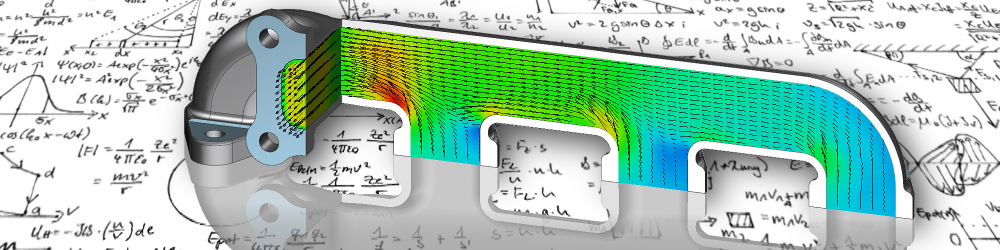•• 1

# Fundamental equations

Depending on the level of abstraction, fluid flow can be described in two different ways. On the one hand on the molecular level through the gas kinetics, on the other hand from a macroscopic point of view through the continuum mechanics.

The Boltzmann equations of gas kinetics can be used to describe all flows of gases and liquids. The fluid is regarded as a set of atoms and molecules that move unordered in space and interact as they approach each other. The characteristic value of the gas kinetics, the Knudsen number, describes the ratio of the average distance travelled between two collisions to the characteristic length of the flow field. If many molecules are present, the probability of collisions increases and the distance travelled is shortened. The Knudsen number decreases accordingly.

For values below 10-2 , the flow state is statistically independent of the details of the molecular movement and can alternatively be described with the approach of continuum mechanics. Apart from special cases, such as vacuum or satellite technology, these requirements are generally met. Molecular flows, which can only be described by gas kinetics, are therefore rare. Since flow simulation based on gas kinetics is generally extremely complex and the necessity for this is the exception, it has no major significance in general engineering practice.

## Continuum mechanic methods

The continuum mechanical description of a flow is done with the Navier-Stokes equations. They apply to all Newtonian fluids and are based on the conservation of impulse, mass and energy. Although this approach is numerically much less complex than gas kinetics due to its macroscopic nature, some processes in the flow are still far too complex to be resolved in detail. This applies in particular to turbulence, multiphase flow and combustion processes. Therefore, the properties and effects of these phenomena are replaced by model equations to reduce the numerical effort. Depending on the level of detail and validity range of the models used, more or less computing time is saved and accuracy is lost in exchange.

Furthermore, it does not make sense to describe all flows with the complete Navier-Stokes equations, since some terms are taken into account in the solution that are only of subordinate significance in the specific application. The Euler equations are obtained by neglecting heat conduction and friction from the Navier-Stokes. Since these only contain first-order derivatives, the gain in computing time is considerable. The Euler equations are used, for example, in the investigation of sound or compression shock waves. Similarly, the Navier-Stokes equations can also be simplified if the conditions for an incompressible flow are present. Since in this case the density is only one substance property, the numerical effort is also reduced here.

Furthermore, it does not make sense to describe all flows with the complete Navier-Stokes equations, since some terms are taken into account in the solution that are only of subordinate significance in the specific application. The Euler equations are obtained by neglecting heat conduction and friction from the Navier-Stokes. Since these only contain first-order derivatives, the gain in computing time is considerable. The Euler equations are used, for example, in the investigation of sound or compression shock waves. Similarly, the Navier-Stokes equations can also be simplified if the conditions for an incompressible flow are present. Since in this case the density is only one substance property, the numerical effort is also reduced here.Warmesberg 24, 9450 Altstätten, Switzerland

+41 79 471 1612